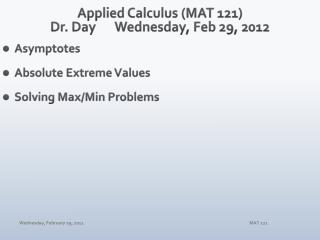# Applied Calculus (MAT 121) Dr. Day Wednes day , Feb 29, 2012 - PowerPoint PPT PresentationDownload PresentationApplied Calculus (MAT 121) Dr. Day Wednes day , Feb 29, 2012

Applied Calculus (MAT 121) Dr. Day Wednes day , Feb 29, 2012Download Presentation## Applied Calculus (MAT 121) Dr. Day Wednes day , Feb 29, 2012

- - - - - - - - - - - - - - - - - - - - - - - - - - - E N D - - - - - - - - - - - - - - - - - - - - - - - - - - -
##### Presentation Transcript

1. Applied Calculus (MAT 121)Dr. Day Wednesday, Feb 29, 2012 • Asymptotes • Absolute Extreme Values • Solving Max/Min Problems MAT 121

2. Absolute Extremes For f(x) = 3x4 + 4x3 + 1, determine its absolute extreme values over the interval [−2,1]. If g(x) = x – 2√x: (i) determine its absolute extreme values over the interval [0,9]. (ii) determine its absolute extreme values for all x. For 2006, operating rate of factories, mines, and utilities in a certain region of the country, expressed as a % of full capacity, is given by the function shown below, where 0 ≤ t ≤ 250 is measured in days. On which of the first 250 days of 2006 was the rate the highest? MAT 121

3. Putting It All Together: Curve Sketching Example: Sketch a graph of the function. Do not show graph on your calculator! MAT 121

4. Solving Max/Min Problems: YOU Define the Functions! • If exactly 200 people sign up for a charter flight, Leisure World Travel Agency charges \$400/person. However, if more than 200 people sign up for the flight (assume this is the case), then each fare is reduced by \$1 for each additional person. Hint: Let x denote the number of passengers above 200. • Determine the revenue function R(x). • Determine how many passengers will result in a maximum revenue for the travel agency. • What is the maximum revenue? • What would be the fare per passenger in this case? MAT 121

5. Solving Max/Min Problems: YOU Define the Functions! • An open-topped box is to be created from a rectangular piece of tin that measures 20 cm by 32 cm. The box is created by removing congruent squares from each corner of the rectangle and then folding up the flaps. • What is the measure of the largest square that could be removed from each corner? • If each cut-out square measures x cm on each side, what is a formula for the volume of the resulting box? • What value of x generates the largest volume? • What would be the volume in this case? MAT 121

6. Assignments WebAssign 4.4 due tonight. 4.5 due tomorrow night. Test #3: Friday, 2 March 2012 MAT 121